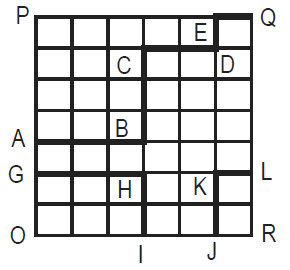# CAT 1991

Instructions

For the following questions answer them individually

Question 1

# Select the set in which the statements are logically related. A. Different hues are obtained from primary colours. B. A rainbow consists of several hues. C. Blue and red can give different hues. D. Red is a primary color. E. Blue can give different hues. F. Red can give different hues.Question 2

# Is it more profitable for Company M to produce Q? I. Product R sells at a price four times that of Q II. One unit of Q requires 2 units of labour, while one unit of R requires 5 units of labour. There is a no other constraint on production.Question 3

# A train started from Station A, developed engine trouble and reached Station B, 40 minutes late. What is the distance between Stations A and B? I. The engine trouble developed after travelling 40 km from Station A and the speed reduced to 1/4th of the original speed. II. The engine trouble developed after travelling 40 km from station A in two hours and the speed reduced to 1/4th of the original speed.Question 4

# What is the value of prime number x? I. $$x^2 + x$$ is a two-digit number greater than 50 II. $$x^3$$ is a three digit number.Question 5

# The average of three unequal quotations for a particular share is Rs.110. If all are quoted in integral values of rupee, does the highest quotation exceed Rs. 129? I. The lowest quotation Rs. 100. II. One of the quotations is Rs. 115.Question 6

# How many people ( from the group surveyed ) read both Indian Express and Times of India? I. Out of total of 200 readers, 100 read Indian Express, 120 read Times of India and 50 read Hindu. II. Out of a total of 200 readers, 100 read Indian Express, 120 reads Times of India and 50 read neither.Question 7

# X says to Y, 'I am 3 times as old as you were 3 years ago'. How old is X? I. Y's age 17 years from now is same as X.s present age. II. X's age nine years from now is 3 times Y.s present age.Question 8

#What is the area under the line GHI-JKL in the given quadrilateral OPQR, knowing that all the small spaces are squares of the same area? I. Length ABCDEQ is greater than or equal to 60. II. Area OPQR is less than or equal to 1512.Question 9

# What is the radius of the circle? I. Ratio of its area to circumference is > 7. II. Diameter of the circle is <=32.Question 10

# What is the time difference between New York and London? I. The departure time at New York is exactly 9.00 a.m local time and the arrival time at London is at 10.00 a.m. local time. II. The flight time is 5 hours.OR